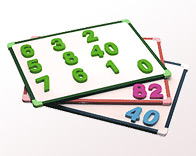# Numbers in a Square

8659This time Geniusbeauty is posting a mathematical brain game. Try to solve this riddle. Place the numbers from 1 to 9 into a 3×3 square so, that the sum of the numbers in each table, raw and diagonal is the same. Please, if you want to find the solution yourself, do not look at the comments of this post, because someone could have already written it there. Good luck!

You will find the solution in the next brain game. You are always welcome to ask questions about the task, in case something is not clear. But sorry, I will not answer leading questions.

The answer to the previous brain game is: turn off all the switches. Wait until the lamps cool. After it turn on two switches and then turn off one of them. If when you come to the closet, the lamp shines, than the needed switch is the switched on one. In case the lamp is off, when you come to the closet, then touch the lamp and check if it is warm or cold. If it is warm, then the needed switch is that one, which was just turned off. If the lamp is cold, then the third one, which was turned off all the time. Congratulations to those, who solved it!

1. hmmm….. I think i’ll wait for your next blog for the answer to this brain game…. only because Im at work at the moment and I left my brains at home :))

2.stargoat

4 9 2
3 5 7
8 1 6

every row = 15
every column = 15
both diagonals = 15

3. Here’s the grid:
2 7 6
9 5 1
4 3 8

Best regards to all,
Chris

4. However, I have found two alternate grids which will work – can you?

5.steve

9 1 5
6 2 7
8 3 4

6.Dwight

Chris, ignoring rotation and reflection there’s only one
3×3 magic square.

7.hj

took 5 minutes at work
9,1,5
3,4,8
6,7,2

8. 8 1 6
3 5 7
4 9 2

This one is easy 🙂

sum: 9 x 10 / 2 = 45 has to be dividen to 3 so on each line sum = 15
9 can’t be on the same line with 3, 6, 7, 8… from there you can do it too 😛

9.Jed Hawk

It’s Magic! (But let’s see if there’s a way to prove them rather than rely on past experience)
The total of all numbers is 45, therefore every row column and diagonal must total 15.

We can conclude that the centre number must be 5.

Why?

If the middle number was 1, we need 4 combinations of 14 from two different numbers(not 1).
But there’s only 9+5, 8+6.
If the middle number was 2, we need 4 combinations of 13 from two different numbers(not 2).
But there’s only 9+4, 8+5, 7+6.
If the middle number was 3, we need 4 combinations of 12 from two different numbers(not 3).
But there’s only 8+4, 7+5.
If the middle number was 4, we need 4 combinations of 11 from two different numbers(not 4).
But there’s only 9+2, 8+3, 6+5.
If the middle number was 6, we need 4 combinations of 9 from two different numbers(not 6).
But there’s only 8+1, 7+2, 5+4.
If the middle number was 7, we need 4 combinations of 8 from two different numbers(not 7).
But there’s only 6+2, 5+3.
If the middle number was 8, we need 4 combinations of 7 from two different numbers(not 8).
But there’s only 6+1, 5+2, 4+3.
If the middle number was 9, we need 4 combinations of 6 from two different numbers(not 9).
But there’s only 5+1, 4+2.

Further, if we stick a number in a corner, we require at least 3 combinations making a total of 15.
From the above, 5 is central, 2,4,6,8 go in the corners SOMEHOW but we must control the placement. Then 1, 3, 7 and 9 go at the sides.
Further, 1 is opposite 9. 2 is opposite 8. 3 is opposite 7. 4 is opposite 6. (using the centre as the pivot)
If we place 9 at any one side, then 8 cannot go in an adjacent corner. It must go in one of the other two. This brings rise to two unique solutions (any others are simply rotations of existing solutions) as Chris has found.

10. Thanks for the logic/mathmetical proof Jed. I have one question for GeniusBeauty. How can people post responses which appear to be prior to posts that have already been made? In future I will annotate my posts with Zulu time.

11. Chris, some of the comments awaite moderation and when they are approved they are listed cronologicaly even if other comments appeared public before them.